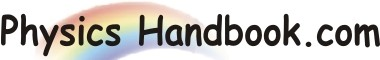HOME TOPICS DEFINITIONS TABLES LAWS INVENTIONS EXPERIMENTS QUIZ VIDEOS
 Stefan-Boltzmann Law The Stefan-Boltzmann law states that the emissive power (E) from a black body is directly proportional to the forth power of its absolute temperature (T). i.e. E = σ x T4 Where, σ  =  Stefan-Boltzmann constant  =  5.67E-8 W / ( m2 K2 ) The law was experimentally discovered by Jozef Stefan ( 1835-1893 ) in 1879 and theoretically derived in the frame of the thermodynamics by Ludwig Boltzmann ( 1844-1906 ) in 1884. The emitted power (E) for a non-black body with emissivity (ε) is E  =  ε x σ x T4